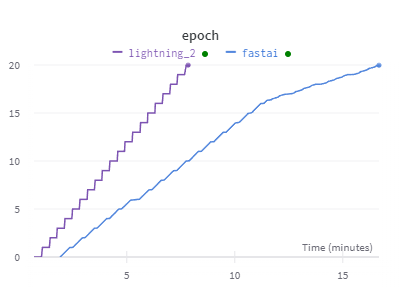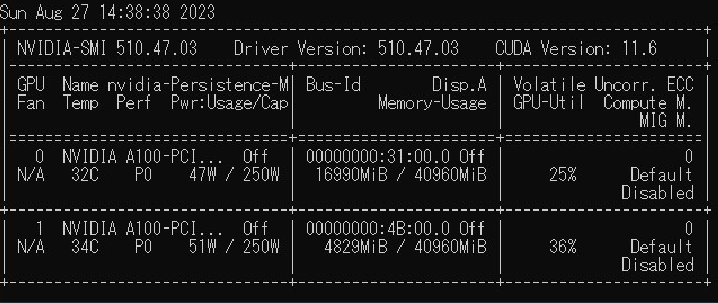# Fastai appears to be slower than PyTorch Lightning on my machine

Hi everyone,

I noticed that Fastai appears to be slower than PyTorch Lightning on my machine.

For instance, the code below is used to classify the CIFAR-10 dataset. It utilizes a ResNet32 model implemented by Timm and was trained for a total of 20 epochs. The dataloaders were created to be as similar as possible.

### Fastai code

``````import os
os. environ['CUDA_VISIBLE_DEVICES'] = '0'

import numpy as np
import pandas as pd
import matplotlib.pyplot as plt
import seaborn as sns
from functools import partial
from fastai.callback.wandb import WandbCallback
from fastai.vision.all import *
import pytorch_lightning as pl
from torch import nn
import timm

import wandb
wandb.init(project="timm", name="fastai")

path = untar_data(URLs.CIFAR)/'train'
test_path = untar_data(URLs.CIFAR)/'test'
device = torch.device("cuda:0")
aug_batch_tfms = [
Flip(p = 0.5),
RandomResizedCropGPU(
size = 32,
min_scale = 0.8,
max_scale =1.0,
ratio = (0.9, 1.1),
p = 0.9
)
]

db = DataBlock(
blocks=(ImageBlock, CategoryBlock),
get_items=get_image_files,
get_y= parent_label,
splitter = RandomSplitter(valid_pct=0.1, seed=42),
n_inp=1,
batch_tfms = aug_batch_tfms + [Normalize.from_stats(*cifar_stats)]#+aug_batch_tfms
)

path,
num_workers=4,
bs = 64
).to(device)

res32 = timm.create_model(
model_name = 'resnet34',
pretrained= False, in_chans = 3, num_classes=10)

learn = Learner(
dls = dls, model = res32, loss_func = CrossEntropyLossFlat(),
cbs = [ShowGraphCallback(), WandbCallback(log_preds_every_epoch = True)],
opt_func=partial(SGD, mom = 0.9, wd = 1e-4),
metrics = accuracy).to_fp16()

# learn.lr_find()

learn.fit_one_cycle(20, 0.001)
``````

### Pytorch lightning code

I am not familiar with PyTorch Lightning. This training framework was written by modifying this toturial.

``````import os
os. environ['CUDA_VISIBLE_DEVICES'] = '1'

import numpy as np
import pandas as pd
import matplotlib.pyplot as plt
import seaborn as sns
from PIL import Image

from lightning.pytorch.loggers import WandbLogger
from pytorch_lightning.callbacks import LearningRateMonitor, ModelCheckpoint
import torch
import torch.nn as nn
import torch.utils.data as data
import torch.optim as optim
# Torchvision
import torchvision
from torchvision.datasets import CIFAR10
from torchvision import transforms
import pytorch_lightning as pl
import timm

import wandb
wandb.init(project="timm", name="lightning")

# Path to the folder where the datasets are/should be downloaded (e.g. CIFAR10)
DATASET_PATH = "../data"
# Path to the folder where the pretrained models are saved
CHECKPOINT_PATH = "../saved_models/tutorial5"
device = torch.device("cuda:0") if torch.cuda.is_available() else torch.device("cpu")

DATA_MEANS = (train_dataset.data / 255.0).mean(axis=(0,1,2))
DATA_STD = (train_dataset.data / 255.0).std(axis=(0,1,2))
print("Data mean", DATA_MEANS)
print("Data std", DATA_STD)

test_transform = transforms.Compose([transforms.ToTensor(),
transforms.Normalize(DATA_MEANS, DATA_STD)
])
# For training, we add some augmentation. Networks are too powerful and would overfit.
train_transform = transforms.Compose([transforms.RandomHorizontalFlip(),
transforms.RandomResizedCrop((32,32), scale=(0.8,1.0), ratio=(0.9,1.1)),
transforms.ToTensor(),
transforms.Normalize(DATA_MEANS, DATA_STD)
])
# Loading the training dataset. We need to split it into a training and validation part
# We need to do a little trick because the validation set should not use the augmentation.
pl.seed_everything(42)
train_set, _ = torch.utils.data.random_split(train_dataset, [45000, 5000])
pl.seed_everything(42)
_, val_set = torch.utils.data.random_split(val_dataset, [45000, 5000])

# We define a set of data loaders that we can use for various purposes later.

class CIFARModule(pl.LightningModule):

def __init__(self, optimizer_name, optimizer_hparams):
"""
Inputs:
model_name - Name of the model/CNN to run. Used for creating the model (see function below)
model_hparams - Hyperparameters for the model, as dictionary.
optimizer_name - Name of the optimizer to use. Currently supported: Adam, SGD
optimizer_hparams - Hyperparameters for the optimizer, as dictionary. This includes learning rate, weight decay, etc.
"""
super().__init__()
# Exports the hyperparameters to a YAML file, and create "self.hparams" namespace
self.save_hyperparameters()
# Create model
self.model = timm.create_model(
model_name = 'resnet34',
pretrained= False, in_chans = 3, num_classes=10)
# Create loss module
self.loss_module = nn.CrossEntropyLoss()
# Example input for visualizing the graph in Tensorboard
self.example_input_array = torch.zeros((1, 3, 32, 32), dtype=torch.float32)

def forward(self, imgs):
# Forward function that is run when visualizing the graph
return self.model(imgs)

def configure_optimizers(self):
# We will support Adam or SGD as optimizers.
# AdamW is Adam with a correct implementation of weight decay (see here for details: https://arxiv.org/pdf/1711.05101.pdf)
self.parameters(), **self.hparams.optimizer_hparams)
elif self.hparams.optimizer_name == "SGD":
optimizer = optim.SGD(self.parameters(), **self.hparams.optimizer_hparams)
else:
assert False, f"Unknown optimizer: \"{self.hparams.optimizer_name}\""

# We will reduce the learning rate by 0.1 after 100 and 150 epochs
# scheduler = optim.lr_scheduler.MultiStepLR(
#     optimizer, milestones=[100, 150], gamma=0.1)
scheduler = optim.lr_scheduler.MultiStepLR(
optimizer, milestones=[10, 15], gamma=0.1)
return [optimizer], [scheduler]

def training_step(self, batch, batch_idx):
# "batch" is the output of the training data loader.
imgs, labels = batch
preds = self.model(imgs)
loss = self.loss_module(preds, labels)
acc = (preds.argmax(dim=-1) == labels).float().mean()

# Logs the accuracy per epoch to tensorboard (weighted average over batches)
self.log('train_acc', acc, on_step=False, on_epoch=True)
self.log('train_loss', loss)
return loss  # Return tensor to call ".backward" on

def validation_step(self, batch, batch_idx):
imgs, labels = batch
preds = self.model(imgs)
loss = self.loss_module(preds, labels)
acc = (preds.argmax(dim=-1) == labels).float().mean()
# By default logs it per epoch (weighted average over batches)
self.log('val_acc', acc)
self.log('valid_loss', loss)

def test_step(self, batch, batch_idx):
imgs, labels = batch
preds = self.model(imgs).argmax(dim=-1)
acc = (labels == preds).float().mean()
# By default logs it per epoch (weighted average over batches), and returns it afterwards
self.log('test_acc', acc)

def train_model(model_name = 'resnet32', save_name=None, **kwargs):
"""
Inputs:
model_name - Name of the model you want to run. Is used to look up the class in "model_dict"
save_name (optional) - If specified, this name will be used for creating the checkpoint and logging directory.
"""
if save_name is None:
save_name = model_name

# Create a PyTorch Lightning trainer with the generation callback
wandb_logger = WandbLogger(project="CIFAR10",wandb_logger = WandbLogger(tags=['accuracy', 'loss']))
trainer = pl.Trainer(default_root_dir=os.path.join(CHECKPOINT_PATH, save_name),                          # Where to save models
accelerator="gpu",                     # We run on a GPU (if possible)
devices=1,                                                                          # How many GPUs/CPUs we want to use (1 is enough for the notebooks)
max_epochs=20,                                                                     # How many epochs to train for if no patience is set
callbacks=[ModelCheckpoint(save_weights_only=True, mode="max", monitor="val_acc"),  # Save the best checkpoint based on the maximum val_acc recorded. Saves only weights and not optimizer
LearningRateMonitor("epoch")],                                           # Log learning rate every epoch
enable_progress_bar=True,
logger=wandb_logger)                                                           # Set to False if you do not want a progress bar
trainer.logger._log_graph = True         # If True, we plot the computation graph in tensorboard
trainer.logger._default_hp_metric = None # Optional logging argument that we don't need

pl.seed_everything(42) # To be reproducable
model = CIFARModule(**kwargs)

# Test best model on validation and test set
result = {"test": test_result["test_acc"], "val": val_result["test_acc"]}

return model, result

resnet_model, resnet_results = train_model(model_name="ResNet",
optimizer_name="SGD",
optimizer_hparams={"lr": 0.1,
"momentum": 0.9,
"weight_decay": 1e-4})
``````

The duration of the training process is depicted in the plot below.Moreover, I noticed that the GPU usage of fastai(CPU0) is lower pytorch lightning(CPU1).I suspected that I wrote the Fastai code incorrectly. However, I’ve been unable to pinpoint the exact issue. Could someone kindly assist me in identifying the cause? Your help would be greatly appreciated.

``````Pytorch lightning version: 2.0.7
Fastai version: 2.7.12
``````

I suspect the primary difference is due to how the images are loaded from disk. You have fastai set to read individual CIFAR images, so lots of slow small random reads. While Lightning indexes images from a single file.

You can use PyTorch dataloaders in fastai. Wrap them in a `DataLoaders`:

``````train_loader = data.DataLoader(train_set, batch_size=64, shuffle=True, drop_last=True, pin_memory=True, num_workers=4)